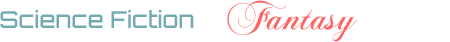## With how many are we ...?

1

(click on this box to dismiss)Q&A for science fiction and fantasy enthusiasts

``````select 0 as reputation,
replace(CONVERT(VARCHAR, CONVERT(MONEY, count(Id)), 1), '.00','') as users
from Users where Reputation >= 0
union
select 100 as reputation,
replace(CONVERT(VARCHAR, CONVERT(MONEY, count(Id)), 1), '.00','') as users
from Users where Reputation > 100
union
select 200 as reputation,
replace(CONVERT(VARCHAR, CONVERT(MONEY, count(Id)), 1), '.00','') as users
from Users where Reputation > 200
union
select 1000 as reputation,
replace(CONVERT(VARCHAR, CONVERT(MONEY, count(Id)), 1), '.00','') as users
from Users where Reputation > 1000
union
select 2000 as reputation,
replace(CONVERT(VARCHAR, CONVERT(MONEY, count(Id)), 1), '.00','') as users
from Users where Reputation > 2000
union
select 10000 as reputation,
replace(CONVERT(VARCHAR, CONVERT(MONEY, count(Id)), 1), '.00','') as users
from Users where Reputation > 10000
union
select 100000 as reputation,
replace(CONVERT(VARCHAR, CONVERT(MONEY, count(Id)), 1), '.00','') as users
from Users where Reputation > 100000
order by reputation``````

### Enter Parameters

Options:
-Hold tight while we fetch your results
:records returned in :time ms:cached